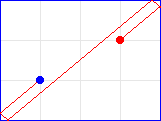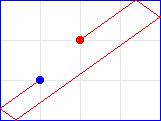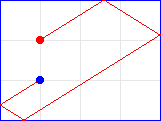### Problem Statement

A "Polyline" is a continuous line composed of one or more line segments. In a rectangle defined by four points (0,0), (a, 0), (a, b), and (0, b), we can draw a polyline starting from the point (x0, y0) to the end point (x1, y1) with at least one intersection point with each of the four edges of the rectangle. Write a class Polyline with a method length that returns the shortest length of such a polyline.

### Definition

 Class: Polyline Method: length Parameters: int, int, int, int, int, int Returns: double Method signature: double length(int a, int b, int x0, int y0, int x1, int y1) (be sure your method is public)

### Notes

-The polyline must not exceed the boundaries of the given rectangle.
-The starting point and ending point may overlap.
-Your return value must have an absolute or relative error less than 1e-9.

### Constraints

-a and b will be between 2 and 200 inclusive.
-x0 and x1 will be between 1 and (a-1) inclusive.
-y0 and y1 will be between 1 and (b-1) inclusive.

### Examples

0)

 `4` `3` `1` `1` `3` `2`
`Returns: 7.810249675906654`1)

 `4` `3` `1` `1` `2` `2`
`Returns: 8.602325267042627`2)

 `4` `3` `1` `1` `1` `2`
`Returns: 9.433981132056603`3)

 `50` `70` `20` `40` `20` `40`
`Returns: 172.04650534085255`
4)

 `98` `200` `78` `32` `35` `174`
`Returns: 299.9549966244937`

#### Problem url:

http://www.topcoder.com/stat?c=problem_statement&pm=4509

#### Problem stats url:

http://www.topcoder.com/tc?module=ProblemDetail&rd=7219&pm=4509

logged

#### Testers:

PabloGilberto , lbackstrom , brett1479

Geometry